# Hidden Metric Spaces

We explore common properties of complex networks in order to find a general mechanism that would support a structural model of the network explaining the connection between network structure and function. Our proposed mechanism relies on the presence of a metric space hidden behind an observable network, referred to as the Hidden Metric Space (HMS). We find that negatively curved hyperbolic HMSes are the most congruent with scale-free strongly clustered real networks such as the Internet.

## HMSes shape the network structure and function

Social experiments have demonstrated that humans can efficiently route through the global acquaintance network topology knowing only some meta-information about their immediate neighbors and the final destination, but lacking the global topology knowledge. In the sociological domain, the network of global acquaintances is a complex network that is both scale-free and strongly clustered. Other networks of social and biological nature, as well as communication networks such as the Internet, exhibit similar characteristics.

To explain these common properties of the Internet and other complex networks, we introduce a hypothesis that nodes in these networks exist in some spaces that are metric and define the observable network topology -- Hidden Metric Spaces. A link between two nodes in the actual topology exists with a certain probability that depends on the distance between these two nodes in the hidden geometry. In the most general way, hidden distances abstract intrinsic similarities between nodes. The assumption that more similar nodes are more likely to be connected makes the connection probability a decreasing function of the hidden distance. In other words, the smaller the distance between two nodes in the hidden metric space, the more likely they are connected in the observable network topology.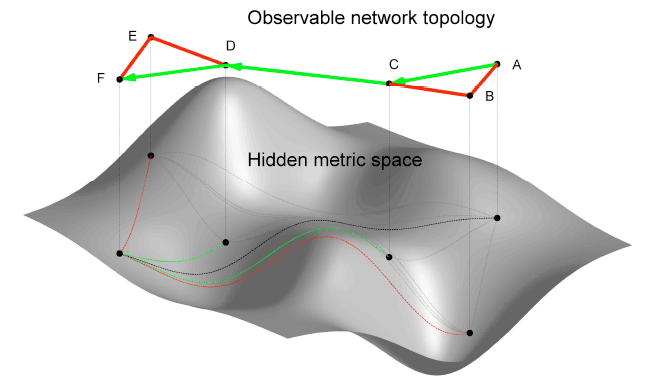Figure 1. Hidden Metric Spaces and Observable Network Topology

Figure 1 illustrates how an underlying HMS influences the topological and functional properties of the graph built on top of it. The observed network topology is closely coupled to the hidden space geometry: if node A is close to node B, and B is close to C in the hidden space, then A and C are necessarily close, too, because of the triangle inequality in the metric space. Therefore, triangle ABC exists in the network topology with high probability, which explains the strong clustering observed in real complex networks. The hidden space also guides the greedy routing process: if node A wants to reach node F (hidden distance AF is the black dashed line), it checks the hidden distances between F and its two neighbours B and C. Distance CF (green dashed line) is smaller than BF (red dashed line), therefore A forwards information to C. Node C then performs similar calculations and selects its neighbor D as the next hop on the path to F. Node D is directly connected to F. The result is path A to C to D to F shown by green edges in the observable topology.

## HMSes explain self-similarity of complex networks

We obtained empirical evidence that metric spaces do underlie real networks. We considered the simplest possible degree-thresholding network renormalization procedure: given a network, obtain its subnetworks by throwing out all nodes of degrees less than a certain threshold as shown in Figure 2.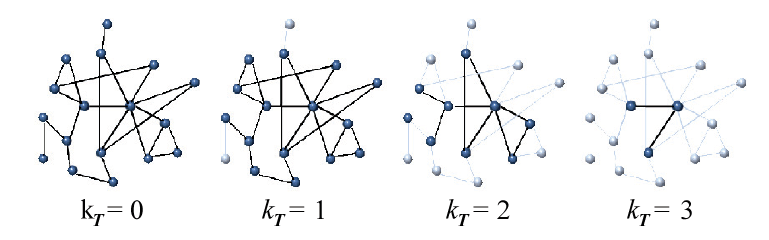Figure 2. Subsequent stages of degree-thresholding network renormalization.

We appled this procedure to real networks and discovered that the degree distribution, degree correlation, and clustering are self-similar: both before and after renormalization, all these characteristics follow the same curves for all considered complex networks, including the Internet. We then randomize the networks by randomly rewiring links such that the degree distribution is preserved. In these randomized networks, the degree distribution and correlations remain self-similar, but clustering does not. Figure 3 illustrates these results for two real networks: the AS-level Internet as seen by the Border Gateway Protocol (BGP) and the Pretty Good Privacy (PGP) social network of mutual trust relationships.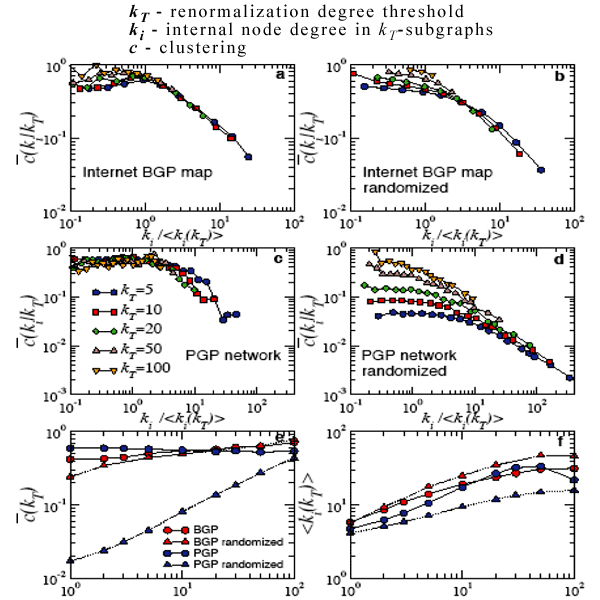Figure 3. Clustering and average degree in renormalized and randomized real networks.

We then introduce the model of scale-free networks with the simplest hidden metric space (a circle) underneath, generate synthetic networks in this model, perform all the same operations described above with these synthetic networks, and find that they exhibit qualitatively the same effects as the real networks (cf Figure 4).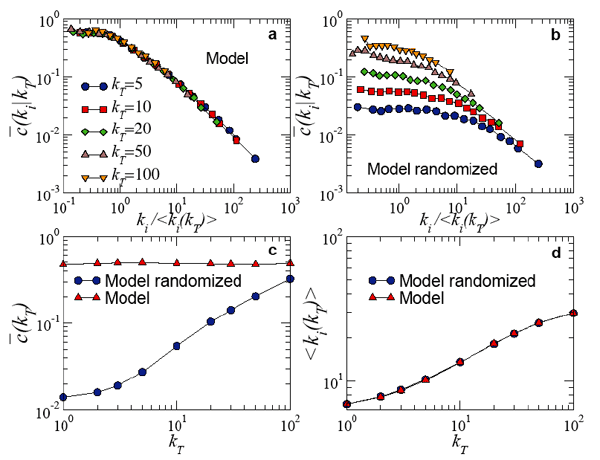Figure 4. Clustering and average degree in renormalized and randomized model networks.

Given the intimate relationship between the triangle inequality in the hidden geometry (transitivity of being close) and clustering in the observed topology (transitivity of being connected), these findings provide a strong evidence that metric spaces do underlie real networks, including the Internet, and are a plausible explanation of their self-similarity.

An interesting and fundamentally important "by-product" of these results is that they offer a new perspective on self-similarity of complex networks. These networks turn out to be self-similar with respect to distance rescaling in the hidden space, because this distance rescaling is equivalent to the degree-thresholding renormalization described above.

Based on findings that complex networks possess this quality of self-similarity, the reconstruction of the hidden metric space underlying the real Internet would lead to a view of network addressing architecture that would be beneficial for efficient and scalable routing.

## k-core decomposition of real and modeled networks

Node coreness is a measure of how deep within the network core the node is located. The k-core is the maximal subgraph induced by nodes that have k or more connections to other nodes in the subgraph; and a node's coreness is k such that the k-core contains the node but the k+1-core does not.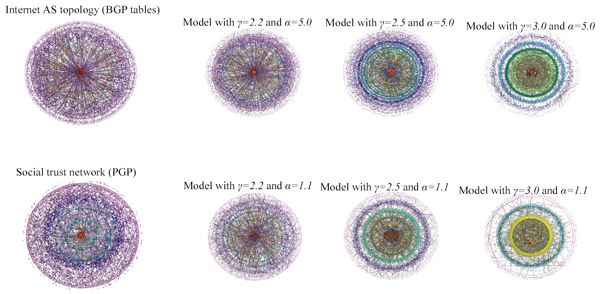Figure 5. k-cores of BGP, PGP, and model networks

In Figure 5, left column depicts k-cores of the same two real networks, BGP and PGP, while the other six images correspond to model networks with different values of power-law exponent γ for degree distribution and strong (α=5.0) or weak (α=1.1) clustering. The network size for all real and modeled cases is approximately 10^4. All nodes are color-coded based on their coreness and size-coded based on their degrees; higher-coreness nodes are also closer to circle centers. There is a remarkable similarity between real networks and modeled networks with γ=2.2 and α=5.0, i.e., with the most navigable parameter values.

## Negative curvature as the main property of HMSes

The interpretation of the HMS-based "geometry-under-topology" model applied to real networks is straightforward: the topology of a real network, i.e., the structure of connections between nodes, is closely coupled with the intrinsic similarities between nodes. The more similar the two nodes, the more likely they are connected. Thus, the geometry underlying a real network is the geometry induced by node similarities.

If this similarity space is taxonomic, i.e., if it allows for a hierarchical classification of nodes, then the hidden geometry is hyperbolic. Hierarchies are (approximately) trees, which embed "almost" isometrically in hyperbolic spaces.

We found that hidden hyperbolic metric spaces explain, simultaneously, the scale-free degree distributions and strong clustering in complex networks. More precisely, the assumption that real networks have some forms of hidden hierarchical organization naturally explains their observed topologies.

Greedy routing mechanisms are efficient in these settings and may offer virtually infinitely scalable routing algorithms for future communication networks. Figure 6 presents illustrations of negative curvature of hyperbolic planes and their similarity to successful and unsuccessful paths in a visualization of a modeled network.Figure 6. Examples of hyperbolic planes.

## Related Objects

See https://catalog.caida.org/paper/2008_self_similarity/ to explore related objects to this document in the CAIDA Resource Catalog.
Published